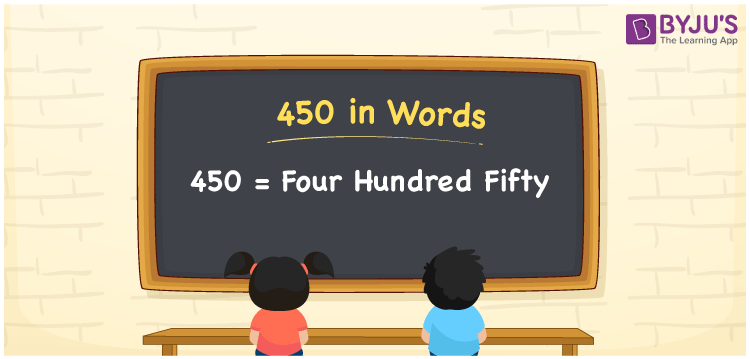# 450 in Words

450 in words is written as “Four hundred fifty”. In Mathematics, 450 is a cardinal number that expresses a quantity or a value or sometimes a currency. If the price of a shirt is Rs.450, then it can be represented as Rupees Four hundred fifty, in English words. Learn more about Numbers In Words at BYJU’S.

 450 in Words Four hundred fifty Four hundred fifty in Numbers 450

## 450 in English Words## How to Write 450 in Words?

The number 450 in words can be written using a place value chart, where we can identify the position of each digit in the number. Since 450 is a three-digit number, thus,

 Hundreds Tens Ones 4 5 0

From the above table,

• 4 is at hundreds’ place
• 5 is at tens’ place
• 0 is at ones’ place

Now, if we read the digits from right to left in the table, it is four hundred fifty.

### Expanded Form of 450

We can write the expanded form as:

4 × Hundred + 5 × Ten + 0 × One

= 4 × 100 + 5 × 10 + 0 × 1

= 450

= Four hundred fifty

450 is a whole number that is succeeded by 449 and preceded by 451. Learn more about the number 450 below:

• 450 in Words – Four hundred fifty
• Is 450 an odd number? – No
• Is 450 an even number? – Yes
• Is 450 a perfect square number? – No
• Is 450 a perfect cube number? – No
• Is 450 a prime number? – No
• Is 450 a composite number? – Yes

## Frequently Asked Questions on 450 in words

Q1

### What is 450 in words?

450 in words is given by Four hundred fifty.
Q2

### What is the rule to write 450 in words?

450 in words is written based on the place values of each digit. In number, 4 is at hundreds place, 5 at tens place and 0 at ones place. So, the number is read as Four hundred fifty in words.
Q3

### What is the value of 450 + 300 in words?

450 + 300 = 750, i.e., Seven hundred fifty.